# Computed Measurements

See it in Epiviz 4

Epiviz exposes a simple expression language that allows users to define new measurements as combinations of existing ones. The expression language is implemented using a JavaScript expression evaluator. This feature can be extremely handy in making simple tweaks in data analysis on the fly, without having to go back and forth to a programming environment.

In the following example we show how to create a couple of simple computed measurements, in order to display the popular MA Plot for colon gene expression of normal vs cancer tissues.

1. Click the Computed Measurements button in the Epiviz toolbar. In the Computed Measurements Dialog, select the data source group whose measurements will be used in creating the new one. Then click on Next to go to the Expression tab. For our example, we choose the `affymetrix_probeset` data source group.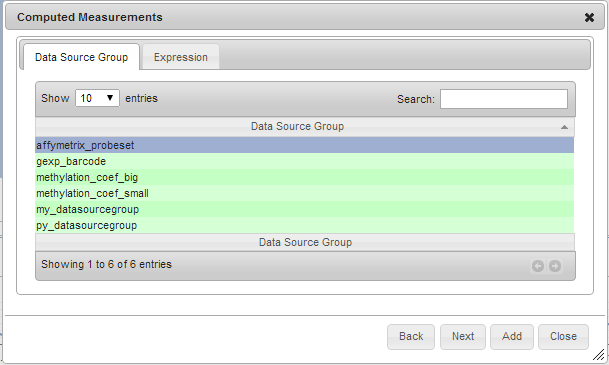1. Fill in the expression, using either the `+` buttons or just writing in the expression text box. Use the measurement identifier next to the measurement name to refer a particular measurement. Here are some example valid expressions:
• `{2} - {3}`
• `({6} + {7}) * 0.5`
• `({0} + {1}) / 2`
• `{1} + random(10) – 5`
• `sin({2})`
• `log({1} - {2})`
• `sqrt({3})`

For our MA Plot Example, we compute two measurements: `M (Colon Expression)` (the difference between `Expression Colon Cancer` and `Expression Colon Normal`) and `A (Colon Expression)` (the average between the two):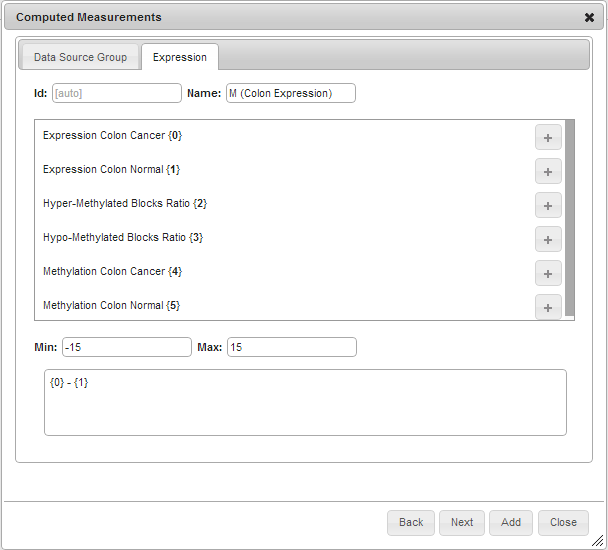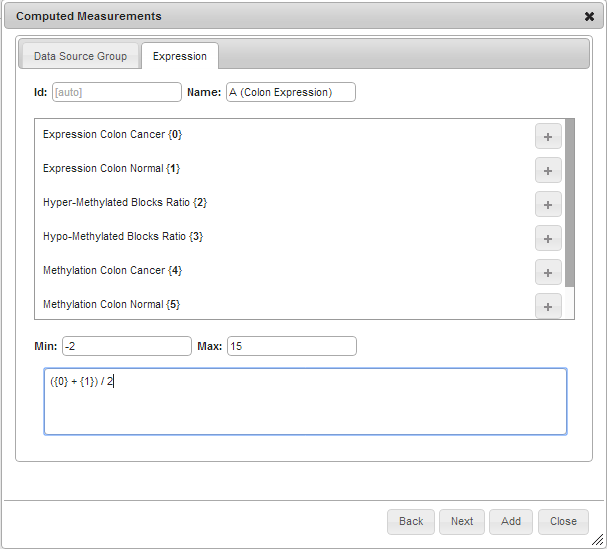1. For each computed measurement, fill in `Name`, `Min` ( optional ) and `Max` ( optional ), and then click Add. When done, click Close.

2. Now add a chart using the newly computed measurement(s). In our example, we choose the Scatter Plot.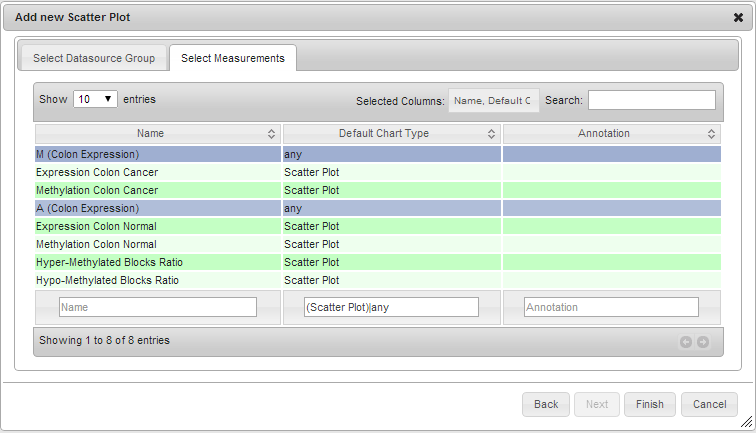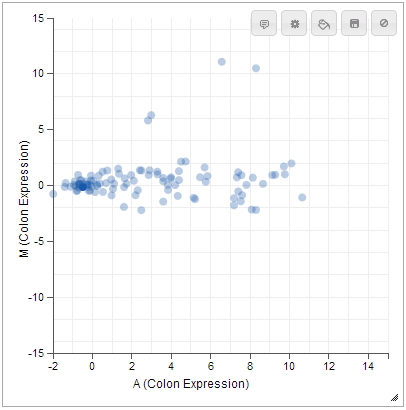See it in Epiviz 4

Description copied from JavaScript Expression Evaluator.

Expression Syntax

The parser accepts a pretty basic grammar. Operators have the normal precidence - `f(x,y,z)` (function calls), `^` (exponentiation), `*`, `/`, and `%` (multiplication, division, and remainder), and finally `+`, `-`, and `||` (addition, subtraction, and string concatenation) - and bind from left to right (yes, even exponentiation it’s simpler that way).

There’s also a `,` (comma) operator that concatenates values into an array. It’s mostly useful for passing arguments to functions, since it doesn’t always behave like you would think with regards to multi-dimensional arrays. If the left value is an array, it pushes the right value onto the end of the array, otherwise, it creates a new array `[left, right]`. This makes it impossible to create an array with another array as it’s first element.

Function operators

The parser has several built-in `functions` that are actually operators. The only difference from an outside point of view, is that they cannot be called with multiple arguments and they are evaluated by the simplify method if their arguments are constant.

Function Description
`sin(x)` Sine of x (x is in radians)
`cos(x)` Cosine of x (x is in radians)
`tan(x)` Tangent of x (x is… well, you know)
`asin(x)` Arc sine of x (in radians)
`acos(x)` Arc cosine of x (in radians)
`atan(x)` Arc tangent of x (in radians)
`sqrt(x)` Square root of x. Result is NaN (Not a Number) if x is negative.
`log(x)` Natural logarithm of x (not base-10). It’s log instead of ln because that’s what JavaScript calls it.
`abs(x)` Absolute value (magnatude) of x
`ceil(x)` Ceiling of x - the smallest integer that’s >= x.
`floor(x)` Floor of x - the largest integer that’s <= x
`round(x)` X, rounded to the nearest integer, using “gradeschool rounding”.
`exp(x)` e^x (exponential/antilogarithm function with base e)

Pre-defined functions

Besides the “operator” functions, there are several pre-defined functions.

Function Description
`random(n)` Get a random number in the range [0, n). If n is zero, or not provided, it defaults to 1.
`fac(n)` n! (factorial of n: “n * (n-1) * (n-2) * … * 2 * 1″)
`min(a,b,...)` Get the smallest (“minimum”) number in the list
`max(a,b,...)` Get the largest (“maximum”) number in the list
`pyt(a, b)` Pythagorean function, i.e. the c in “c2 = a2 + b2”
`pow(x, y)` x^y. This is exactly the same as “x^y”. It’s just provided since it’s in the Math object from JavaScript
`atan2(y, x)` arc tangent of x/y. i.e. the angle between (0, 0) and (x, y) in radians.